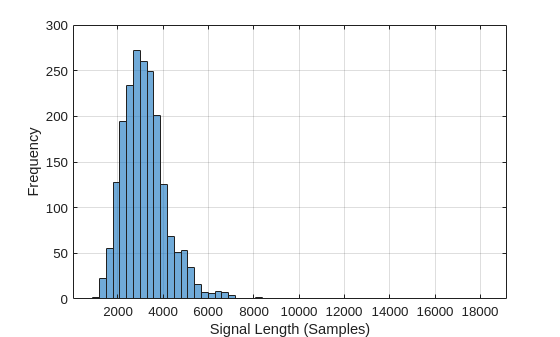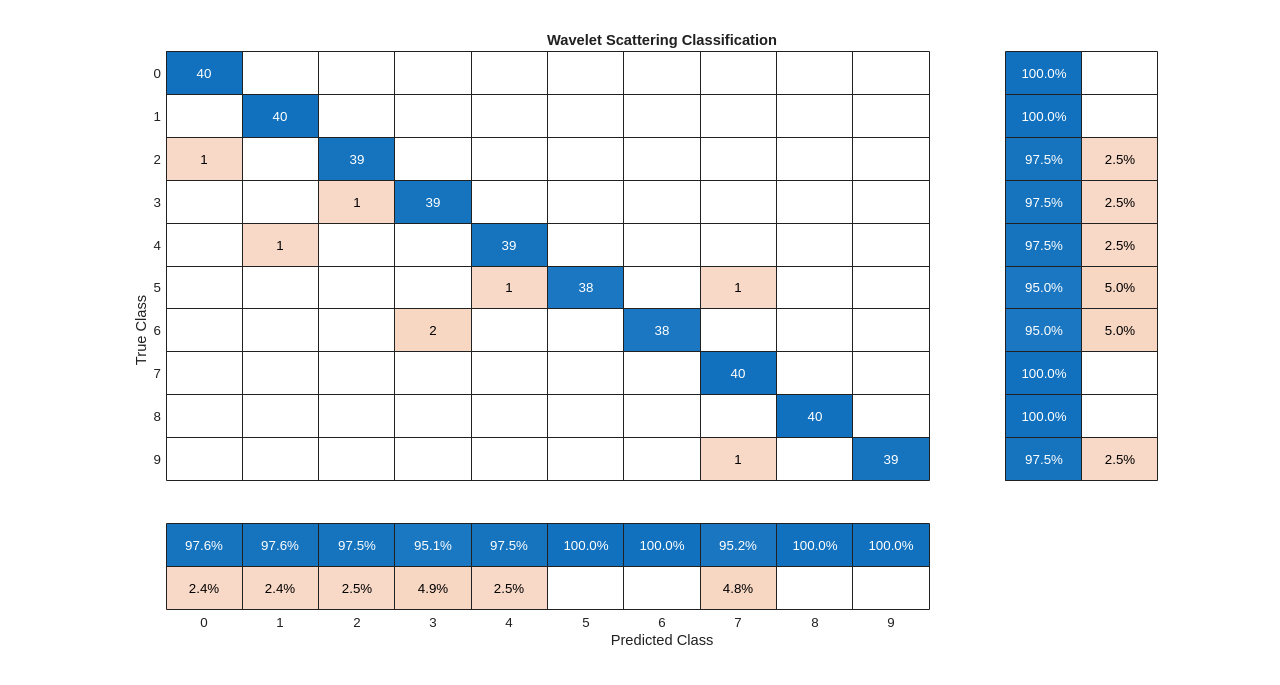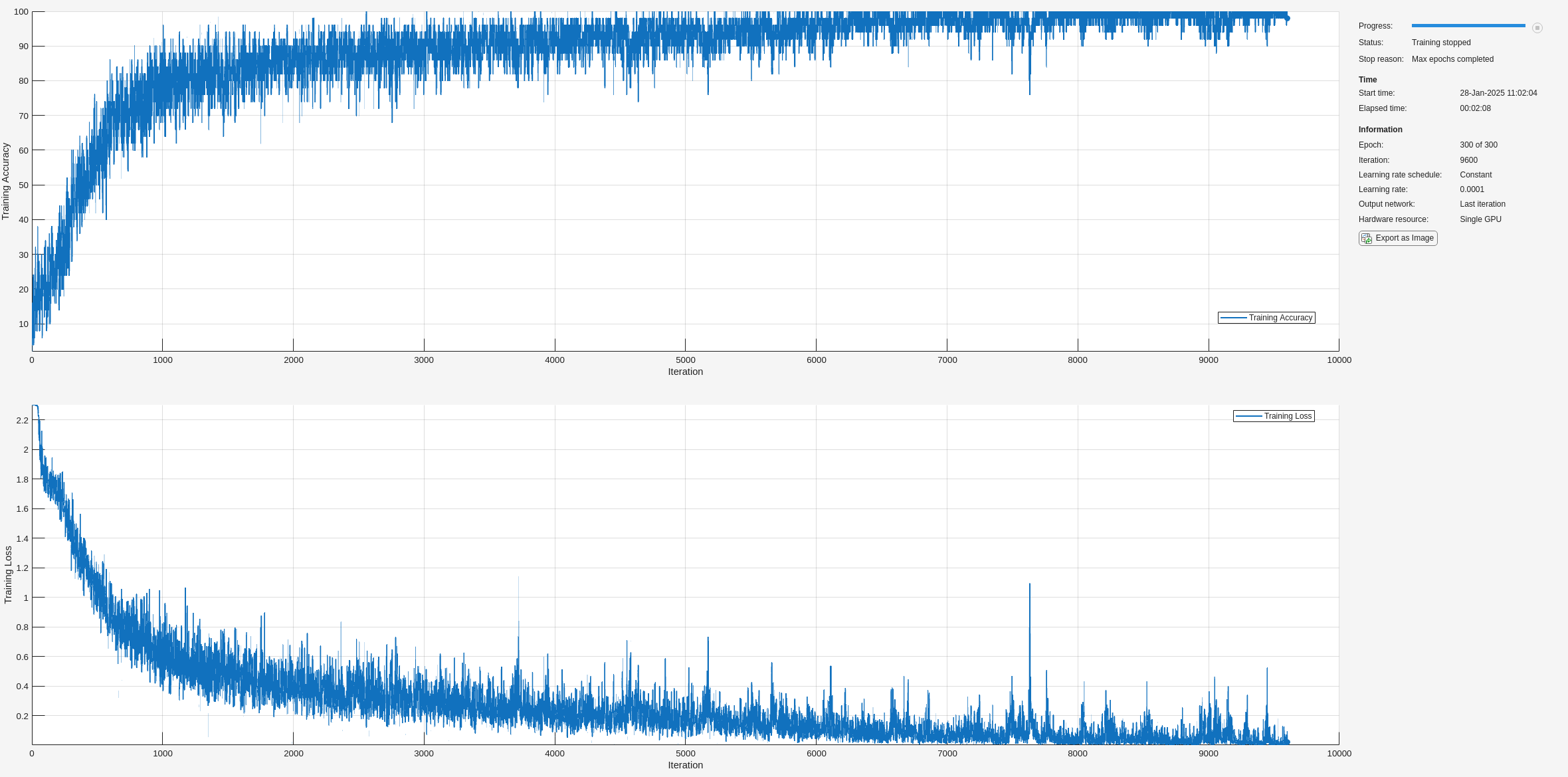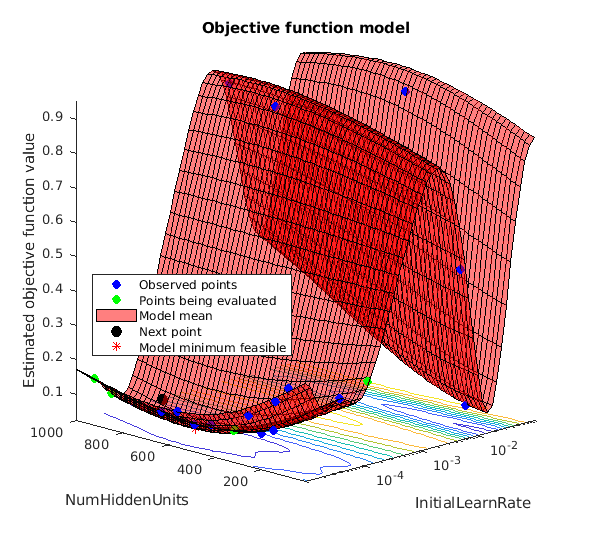## Spoken Digit Recognition with Wavelet Scattering and Deep Learning

This example shows how to classify spoken digits using wavelet time scattering paired with a support vector machine and a deep convolutional network based on mel-frequency spectrograms.

### Data

Clone or download the Free Spoken Digit Dataset (FSDD), available at https://github.com/Jakobovski/free-spoken-digit-dataset. FSDD is an open data set, which means that it can grow over time. This example uses the version committed on 01/29/2019 which consists of 2000 recordings of the English digits 0 through 9 obtained from four speakers. Two of the speakers in this version are native speakers of American English and two speakers are nonnative speakers of English with a Belgium French and German accent. The data is sampled at 8000 Hz.

Use `audioDatastore` to manage data access and ensure the random division of the recordings into training and test sets. Set the `location` property to the location of the FSDD recordings folder on your computer, for example:

```pathToRecordingsFolder = '/home/user/free-spoken-digit-dataset/recordings'; location = pathToRecordingsFolder;```

Point `audioDatastore `to that location.

`ads = audioDatastore(location);`

The helper function `helpergenLabels`, defined at the end of this example, creates a categorical array of labels from the FSDD files. List the classes and the number of examples in each class.

```ads.Labels = helpergenLabels(ads); summary(ads.Labels)```
``` 1 200 0 200 2 200 3 200 4 200 5 200 6 200 7 200 8 200 9 200 ```

The FSDD dataset consists of 10 balanced classes with 200 recordings each. The recordings in the FSDD are not of equal duration. Because the FSDD is not prohibitively large, read through the FSDD files and construct a histogram of the signal lengths.

```LenSig = zeros(numel(ads.Files),1); nr = 1; while hasdata(ads) digit = read(ads); LenSig(nr) = numel(digit); nr = nr+1; end reset(ads) histogram(LenSig) grid on xlabel('Signal Length (Samples)') ylabel('Frequency')```The histogram shows that the distribution of recording lengths is positively skewed. For classification, this example uses a common signal length of 8192 samples. The value of 8192 was chosen to conservatively ensure that truncating longer recordings did not affect (cut off) the speech content. If the signal is greater than 8192 samples, or 1.024 seconds, in length, we truncate the recording to 8192 samples. If the signal is less than 8192 samples in length, we symmetrically prepad and postpad the signal with zeros out to a length of 8192 samples.

### Wavelet Time Scattering

Use `waveletScattering` to create a wavelet time scattering framework using an invariant scale of 0.22 seconds. Because we will create feature vectors by averaging the scattering transform over all time samples, set the `OversamplingFactor` to 2, resulting in a four-fold increase in the number of scattering coefficients for each path with respect to the critically downsampled value.

```sf = waveletScattering('SignalLength',8192,'InvarianceScale',0.22,... 'SamplingFrequency',8000,'OversamplingFactor',2);```

Split the FSDD into training and test sets. Allocate 80% of the data to the training set and retain 20% for the test set. The training data is for training the classifier based on the scattering transform. The test data is for validating the model.

```rng default; ads = shuffle(ads); [adsTrain,adsTest] = splitEachLabel(ads,0.8); countEachLabel(adsTrain)```
```ans=10×2 table Label Count _____ _____ 0 160 1 160 2 160 3 160 4 160 5 160 6 160 7 160 8 160 9 160 ```
`countEachLabel(adsTest)`
```ans=10×2 table Label Count _____ _____ 0 40 1 40 2 40 3 40 4 40 5 40 6 40 7 40 8 40 9 40 ```

Form a 8192-by-1600 matrix where each column is a spoken-digit recording. The helper function `helperReadSPData` truncates or pads the data to length 8192 and normalizes each record by its maximum value.

```Xtrain = []; scatds_Train = transform(adsTrain,@(x)helperReadSPData(x)); while hasdata(scatds_Train) smat = read(scatds_Train); Xtrain = cat(2,Xtrain,smat); end```

Repeat the process for the held-out test set. The resulting matrix is 8192-by-400.

```Xtest = []; scatds_Test = transform(adsTest,@(x)helperReadSPData(x)); while hasdata(scatds_Test) smat = read(scatds_Test); Xtest = cat(2,Xtest,smat); end```

Apply the wavelet scattering transform to the training and test sets.

```Strain = sf.featureMatrix(Xtrain); Stest = sf.featureMatrix(Xtest);```

Obtain the mean scattering features for the training and test sets. Exclude the 0-th order scattering coefficients.

```TrainFeatures = Strain(2:end,:,:); TrainFeatures = squeeze(mean(TrainFeatures,2))'; TestFeatures = Stest(2:end,:,:); TestFeatures = squeeze(mean(TestFeatures,2))';```

This example uses a support vector machine (SVM) classifier with the scattering features. The SVM uses a quadratic polynomial kernel.

```template = templateSVM(... 'KernelFunction', 'polynomial', ... 'PolynomialOrder', 2, ... 'KernelScale', 'auto', ... 'BoxConstraint', 1, ... 'Standardize', true); classificationSVM = fitcecoc(... TrainFeatures, ... adsTrain.Labels, ... 'Learners', template, ... 'Coding', 'onevsone', ... 'ClassNames', categorical({'1'; '0'; '2'; '3'; '4'; '5'; '6'; '7'; '8'; '9'}));```

Use k-fold cross-validation to predict the generalization accuracy of the model based on the training data. Split the training set into five groups.

```partitionedModel = crossval(classificationSVM, 'KFold', 5); [validationPredictions, validationScores] = kfoldPredict(partitionedModel); validationAccuracy = (1 - kfoldLoss(partitionedModel, 'LossFun', 'ClassifError'))*100```
```validationAccuracy = 96.8750 ```

The estimated generalization accuracy is approximately 97%. Use the trained SVM to predict the spoken-digit classes in the held-out test set.

```predLabels = predict(classificationSVM,TestFeatures); testAccuracy = sum(predLabels==adsTest.Labels)/numel(predLabels)*100```
```testAccuracy = 98 ```

Summarize the performance of the model on the test set with a confusion chart. Display the precision and recall for each class by using column and row summaries. The table at the bottom of the confusion chart shows the precision values for each class. The table to the right of the confusion chart shows the recall values.

```figure('Units','normalized','Position',[0.2 0.2 0.5 0.5]); ccscat = confusionchart(adsTest.Labels,predLabels); ccscat.Title = 'Wavelet Scattering Classification'; ccscat.ColumnSummary = 'column-normalized'; ccscat.RowSummary = 'row-normalized';```The scattering transform coupled with a SVM classifier classifies the spoken digits in the test set with an accuracy percentage of 98, or an error rate of 2%.

### Deep Convolutional Network Using Mel-Frequency Spectrograms

As another approach to the task of spoken digit recognition, use a deep convolutional neural network (DCNN) based on mel-frequency spectrograms to classify the FSDD data set. Use the same signal truncation/padding procedure as in the scattering transform. Similarly, normalize each recording by dividing each signal sample by the maximum absolute value. For consistency, use the same training and test sets as for the scattering transform.

Set the parameters for the mel-frequency spectrograms. Use the same window, or frame, duration as in the scattering transform, 0.22 seconds. Set the hop between windows to 10 ms. Use 40 frequency bands.

```segmentDuration = 8192*(1/8000); frameDuration = 0.22; hopDuration = 0.01; numBands = 40;```

Reset the training and test datastores.

```reset(adsTrain); reset(adsTest);```

The helper function `helperspeechSpectrograms`, defined at the end of this example, uses `melSpectrogram` to obtain the mel-frequency spectrogram after standardizing the recording length and normalizing the amplitude. Use the logarithm of the mel-frequency spectrograms as the inputs to the DCNN. To avoid taking the logarithm of zero, add a small epsilon to each element.

```epsil = 1e-6; XTrain = helperspeechSpectrograms(adsTrain,segmentDuration,frameDuration,hopDuration,numBands);```
```Computing speech spectrograms... Processed 500 files out of 1600 Processed 1000 files out of 1600 Processed 1500 files out of 1600 ...done ```
```XTrain = log10(XTrain + epsil); XTest = helperspeechSpectrograms(adsTest,segmentDuration,frameDuration,hopDuration,numBands);```
```Computing speech spectrograms... ...done ```
```XTest = log10(XTest + epsil); YTrain = adsTrain.Labels; YTest = adsTest.Labels;```

### Define DCNN Architecture

Construct a small DCNN as an array of layers. Use convolutional and batch normalization layers, and downsample the feature maps using max pooling layers. To reduce the possibility of the network memorizing specific features of the training data, add a small amount of dropout to the input to the last fully connected layer.

```sz = size(XTrain); specSize = sz(1:2); imageSize = [specSize 1]; numClasses = numel(categories(YTrain)); dropoutProb = 0.2; numF = 12; layers = [ imageInputLayer(imageSize) convolution2dLayer(5,numF,'Padding','same') batchNormalizationLayer reluLayer maxPooling2dLayer(3,'Stride',2,'Padding','same') convolution2dLayer(3,2*numF,'Padding','same') batchNormalizationLayer reluLayer maxPooling2dLayer(3,'Stride',2,'Padding','same') convolution2dLayer(3,4*numF,'Padding','same') batchNormalizationLayer reluLayer maxPooling2dLayer(3,'Stride',2,'Padding','same') convolution2dLayer(3,4*numF,'Padding','same') batchNormalizationLayer reluLayer convolution2dLayer(3,4*numF,'Padding','same') batchNormalizationLayer reluLayer maxPooling2dLayer(2) dropoutLayer(dropoutProb) fullyConnectedLayer(numClasses) softmaxLayer classificationLayer('Classes',categories(YTrain)); ];```

Set the hyperparameters to use in training the network. Use a mini-batch size of 50 and a learning rate of 1e-4. Specify Adam optimization. Because the amount of data in this example is relatively small, set the execution environment to `'cpu'` for reproducibility. You can also train the network on an available GPU by setting the execution environment to either `'gpu'` or `'auto'`. For more information, see `trainingOptions`.

```miniBatchSize = 50; options = trainingOptions('adam', ... 'InitialLearnRate',1e-4, ... 'MaxEpochs',30, ... 'MiniBatchSize',miniBatchSize, ... 'Shuffle','every-epoch', ... 'Plots','training-progress', ... 'Verbose',false, ... 'ExecutionEnvironment','cpu');```

Train the network.

`trainedNet = trainNetwork(XTrain,YTrain,layers,options);`Use the trained network to predict the digit labels for the test set.

```[Ypredicted,probs] = classify(trainedNet,XTest,'ExecutionEnvironment','CPU'); cnnAccuracy = sum(Ypredicted==YTest)/numel(YTest)*100```
```cnnAccuracy = 97.7500 ```

Summarize performance of the trained network on the test set with a confusion chart. Display the precision and recall for each class by using column and row summaries. The table at the bottom of the confusion chart shows the precision values. The table to the right of the confusion chart shows the recall values.

```figure('Units','normalized','Position',[0.2 0.2 0.5 0.5]); ccDCNN = confusionchart(YTest,Ypredicted); ccDCNN.Title = 'Confusion Chart for DCNN'; ccDCNN.ColumnSummary = 'column-normalized'; ccDCNN.RowSummary = 'row-normalized';```The DCNN using mel-frequency spectrograms as inputs classifies the spoken digits in the test set with an accuracy rate of approximately 98% as well.

### Summary

This example shows how to use two different approaches for classifying spoken digits in the FSDD. The goal of the example is simply to demonstrate how to use MathWorks™ tools to approach the problem in two fundamentally different but complementary ways. Both workflows use `audioDatastore` to manage flow of data from disk and ensure proper randomization.

One learning approach uses wavelet time scattering paired with a support vector machine classifier. The other learning approach uses mel-frequency spectrograms as inputs to a DCNN. Both approaches perform well on the test set. This example is not intended as a direct comparison between the two approaches. With both methods, you can try different hyperparameters and architectures, which can significantly affect the results. In the case of the mel-frequency spectrogram approach, you can experiment with different parameterizations of the mel-frequency spectrogram as well as changes to the DCNN layers, including adding layers. An additional strategy that is useful in deep learning with small training sets like this version of the FSDD is to use data augmentation. How manipulations affect class is not always known, so data augmentation is not always feasible. However, for speech, established data augmentation strategies are available through `audioDataAugmenter`.

In the case of wavelet time scattering, there are also a number of modifications you can try. For example, you can change the invariant scale of the transform, vary the number of wavelet filters per filter bank, and try different classifiers.

### Appendix: Helper Functions

```function Labels = helpergenLabels(ads) % This function is only for use in the % "Spoken Digit Recognition with Wavelet Scattering and Deep Learning" % example. It may change or be removed in a future release. Labels = categorical(numel(ads.Files),1); expression = "[0-9]+_"; for nf = 1:numel(ads.Files) idx = regexp(ads.Files{nf},expression); Labels(nf) = categorical(str2double(ads.Files{nf}(idx))); end end```

```function x = helperReadSPData(x) % This function is only for use Wavelet Toolbox examples. It may change or % be removed in a future release. N = numel(x); if N > 8192 x = x(1:8192); elseif N < 8192 pad = 8192-N; prepad = floor(pad/2); postpad = ceil(pad/2); x = [zeros(prepad,1) ; x ; zeros(postpad,1)]; end x = x./max(abs(x)); end```

```function X = helperspeechSpectrograms(ads,segmentDuration,frameDuration,hopDuration,numBands) % This function is only for use in the % "Spoken Digit Recognition with Wavelet Scattering and Deep Learning" % example. It may change or be removed in a future release. % % helperspeechSpectrograms(ads,segmentDuration,frameDuration,hopDuration,numBands) % computes speech spectrograms for the files in the datastore ads. % segmentDuration is the total duration of the speech clips (in seconds), % frameDuration the duration of each spectrogram frame, hopDuration the % time shift between each spectrogram frame, and numBands the number of % frequency bands. disp("Computing speech spectrograms..."); numHops = ceil((segmentDuration - frameDuration)/hopDuration); numFiles = length(ads.Files); X = zeros([numBands,numHops,1,numFiles],'single'); for i = 1:numFiles [x,info] = read(ads); x = normalizeAndResize(x); fs = info.SampleRate; frameLength = round(frameDuration*fs); hopLength = round(hopDuration*fs); spec = melSpectrogram(x,fs, ... 'WindowLength',frameLength, ... 'OverlapLength',frameLength - hopLength, ... 'FFTLength',2048, ... 'NumBands',numBands, ... 'FrequencyRange',[50,4000]); % If the spectrogram is less wide than numHops, then put spectrogram in % the middle of X. w = size(spec,2); left = floor((numHops-w)/2)+1; ind = left:left+w-1; X(:,ind,1,i) = spec; if mod(i,500) == 0 disp("Processed " + i + " files out of " + numFiles) end end disp("...done"); end %-------------------------------------------------------------------------- function x = normalizeAndResize(x) % This function is only for use in the % "Spoken Digit Recognition with Wavelet Scattering and Deep Learning" % example. It may change or be removed in a future release. N = numel(x); if N > 8192 x = x(1:8192); elseif N < 8192 pad = 8192-N; prepad = floor(pad/2); postpad = ceil(pad/2); x = [zeros(prepad,1) ; x ; zeros(postpad,1)]; end x = x./max(abs(x)); end```